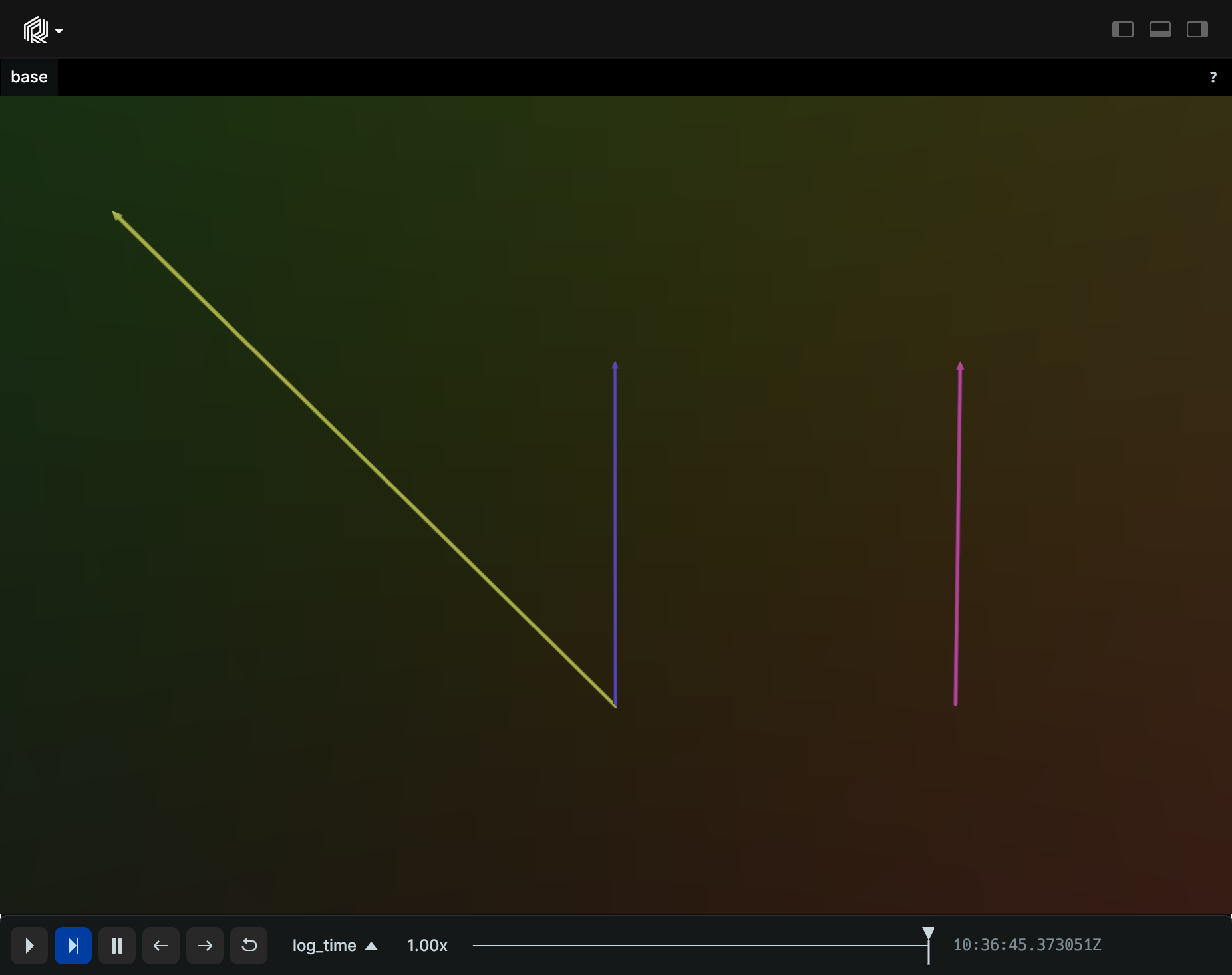# Transform3D

`Transform3D` represents an affine 3D transformation between an entity and it's parent space in the entity hierarchy. Read more about spaces and transforms here

## Components and APIs

Primary component: `transform3d`

Python APIs: log_transform3d

Rust API: Transform3D

## Simple Example

``````"""Log different transforms between three arrows."""
import rerun as rr

rr.init("rerun_example_transform", spawn=True)

origin = [0, 0, 0]
base_vector = [0, 1, 0]

rr.log_arrow("base", origin=origin, vector=base_vector)

rr.log_transform3d("base/translated", rr.Translation3D([1, 0, 0]))

rr.log_arrow("base/translated", origin=origin, vector=base_vector)

rr.log_transform3d(
"base/rotated_scaled",
rr.TranslationRotationScale3D(rotation=rr.RotationAxisAngle(axis=[0, 0, 1], radians=3.14 / 4), scale=rr.Scale3D(2)),
)

rr.log_arrow("base/rotated_scaled", origin=origin, vector=base_vector)
``````